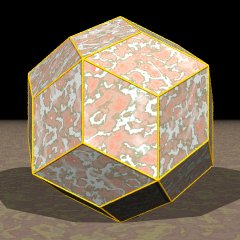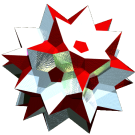Acronym rhote (old: rattic) Name rhombic triacontahedron,terminally chamfered dodecahedron,terminally chamfered icosahedron,surtegmated dodecahedron,surtegmated icosahedron `© ©` Inradius sqrt[(5+2 sqrt(5))/5] = 1.376382 Vertex figure [r5], [R3] Dual id Dihedral angles (at margins) between {(r,R)2} and {(r,R)2}:   144° ExternallinksThe rhombs {(r,R)2} have vertex angles r = arccos(1/sqrt(5)) = 63.434949° resp. R = arccos(-1/sqrt(5)) = 116.565051°. Esp. rr : RR = (1+sqrt(5))/2.

All a and b edges, provided in the below description, only qualify as pseudo edges wrt. the full polyhedron. Edge size used here is rR = x = 1.

Incidence matrix according to Dynkin symbol

```o3m5o =
ao3oo5ob&#zx   → height = 0,
a = rr = 2 sqrt[(5+sqrt(5))/10] = 1.701302,
b = RR = 2 sqrt[(5-sqrt(5))/10] = 1.051462

o.3o.5o.     | 12  * |  5 |  5  [r5]
.o3.o5.o     |  * 20 |  3 |  3  [R3]
-------------+-------+----+---
oo3oo5oo&#x  |  1  1 | 60 |  2
-------------+-------+----+---
ao .. ob&#zx |  2  2 |  4 | 30  {(r,R)2}
```